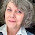## Monday, October 17, 2016

### Divisibility Song - Easily Memorize Divisibility Rules!Learn Divisibility Rules by Singing them!
It's great to have tricks for determining divisibility, but they're not much use if you can't remember them! This is a song I made up ("wrote" seems too strong a word!) for my little girl to help her remember all this stuff. I tried to avoid terms like "divisor" and "dividend" because they're hard to remember, too! (and a memory song is only useful if you can figure out what it means when you go to do the math!)

In our home school, we do one type of memory song per day: Religion on Monday, Math Songs on Tuesday, History & Geography on Wednesday, and Science on Friday (yes, we only schedule 4 days of structured school per week). Singing this song every Tuesday makes those math problems a snap when they arise.

I omitted the tips for 7 & 11, as they are rather ungainly and time-consuming; it seems easier to just "do the math" and figure those out the old-fashioned way!

If you like this post, also check out

I got these tips from the internet, relying heavily on this chart from the Contented at Home blog, which I also encourage downloading as a great home school (or traditional school) resource.

Free Disivibility Chart

Here's a little video of the tune being played by an adorable little girl, in case you have trouble recalling it:

Divisibility Song
To the Tune of "Frere Jaques"
or "Are You Sleeping, Brother John"
(if you need a reminder of the tune, click the video, above)

If a number
ends in zero
then you know
that you can
easily divide it
by 2, 5, or 10
It's a Snap!
It's a Snap!

You can divide
numbers by two
if they're even
that's the test
you can divide numbers
by two if they're even
that's the test! That's the test!

You can divide
numbers by three
if their sum+
divides by three
You can divide
numbers
by three if their sum
divides by three
Yes you can!

You can divide
numbers by four
if the last
two digits
can divide by four
can divide by four
then you know
it will work!

You can divide
numbers by five
if their last
digit is
either 5 or zero
either 5 or zero
then you can divide by 5

You can divide
numbers by six
if you can
divide them
by both 2 and 3
by both 2 and 3
then you can
divide by 6!

You can divide
numbers by 8
if their last
three digits
can divide by 8
can divide by 8
then you know
it will work

You can divide
numbers by 9
if the sum+
divides by 9
you can divide numbers
by 9 if the sum
divides by 9
Yes you can!

You can divide
numbers by 10
if they end
in zero
You can divide numbers
by 10 if they end
in zero, in zero!

You can divide
numbers by twelve
if you can
divide them
by both 3 and 4
by both 3 and 4
then you can
divide by 12!

in division
Multiply
by the left number *
(or the bottom number) **
then you'll know
that it's right!
__3___
*When formatting Division questions like this: 5 ) 15      then it's the Left Number
** When formatting Division Questions as is common in Algebra  15 =3  then it's the bottom number.
5

+A "sum" of a number means that you add up all the digits of a number till you get a single digit - that's the number's sum. For instance, the sum of 123 is 1+2+3= 6. And the sum of 12345 is 1+2+3+4+5=15  THEN 1+5 =6, So the sum of 12345 is also 6.

If you like this, you might want to check out my other Homeschooling Posts

Home School for Cheap or Free: Why Greek is Better than Latin!
Home School for Cheap or Free: Greek Reading
Home School for Cheap or Free: Greek Conversation
Teaching Math: Home School for Cheap or Free
Teaching Music: Homeschool for Cheap or Free
Teach Reading with Confidence: Homeschool for Chea...

This is being shared on
Mix it Up Monday
Merry Monday
The Art of Homemaking Mondays
Modest Mondays
What'd You Do This Weekend?
Literacy Musing Mondays
Together on Tuesdays
Tuesdays with a Twist
Monday Mish Mash
Teaching What is Good
Wake Up Wednesday
Coffee & Conversation
Creative Spark
This is How We Roll
Think Tank Thursday
Friday Favorites
Homeschool Memos
Home Matters
Faith Filled Friday
Pretty Pintastic

1.What a great idea! Thanks for sharing at Together on Tuesdays :)

1.Thanks so much - glad you liked it! Thanks for hosting! : )

Due to an exceptionally high current spam comment problem, I have turned off comments for the time being.